# 6- GENERAL PRINCIPLE AND PROCESSES OF ISOLATION OF ELEMENTS

Here we are going through the ncert solutions for class 12 chemistry chapter 6 – General principle and processes of isolation of elements so before going through the ncert solutions make sure to go through the textbook that helps you to understand the solutions more easily

# ​​ ​​ ​​ ​​ ​​ ​​ ​​ ​​ ​​ ​​ ​​ ​​ ​​ ​​ ​​ ​​ ​​ ​​ ​​ ​​ ​​ ​​ ​​ ​​ ​​ ​​ ​​ ​​ ​​ ​​ ​​ ​​ ​​ ​​ ​​ ​​ ​​ ​​ ​​ ​​ ​​ ​​ ​​ ​​ ​​ ​​ ​​ ​​ ​​ ​​ ​​ ​​ ​​ ​​ ​​​​ INTEXT ANSWERS

#### Question 6.1:

Which of the ores mentioned in Table 6.1 can be concentrated by magnetic separation method?

If the ore or the gangue can be attracted by the magnetic field, then the ore can be concentrated by the process of magnetic separation. Among the ores mentioned in table 6.1, the ores of iron such as haematite (Fe2O3), magnetite (Fe3O4), siderite (FeCO3), and iron pyrites (FeS2) can be separated by the process of magnetic separation.

#### Question 6.2:

What is the significance of leaching in the extraction of aluminium?

In the extraction of aluminium, the significance of leaching is to concentrate pure alumina (Al2O3) from bauxite ore.

Bauxite usually contains silica, iron oxide, and titanium oxide as impurities. In the process of leaching, alumina is concentrated by digesting the powdered ore with a concentrated solution of NaOH at 473-523 K and 35-36 bar. Under these conditions, alumina (Al2O3) dissolves as sodium meta-aluminate and silica (SiO2) dissolves as sodium silicate leaving the impurities behind.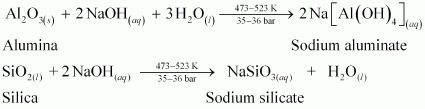The impurities are then filtered and the solution is neutralized by passing CO2 gas. In this process, hydrated Al2O3 gets precipitated and sodium silicate remains in the solution. Precipitation is induced by seeding the solution with freshly prepared samples of hydrated Al2O3.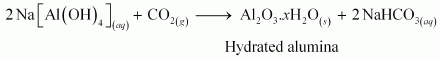Hydrated alumina thus obtained is filtered, dried, and heated to give back pure alumina (Al2O3).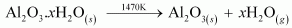Question 6.3:

The reaction,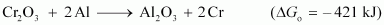is thermodynamically feasible as is apparent from the Gibbs energy value.

Why does it not take place at room temperature?

The change in Gibbs energy is related to the equilibrium constant, K as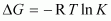.

At room temperature, all reactants and products of the given reaction are in the solid state. As a result, equilibrium does not exist between the reactants and the products. Hence, the reaction does not take place at room temperature. However, at a higher temperature, chromium melts and the reaction takes place.

We also know that according to the equation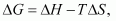Increasing the temperature increases the value of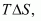making the value of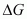more and more negative. Therefore, the reaction becomes more and more feasible as the temperature is increased.

#### Question 6.4:

Is it true that under certain conditions, Mg can reduce SiO2 and Si can reduce MgO? What are those conditions?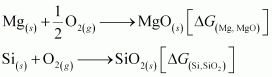The temperature range in which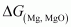is lesser than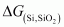, Mg can reduce SiO2 to Si.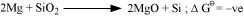On the other hand, the temperatures range in whichis less than, Si can reduce MgO to Mg.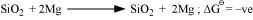The temperature at which ΔfG curves of these two substances intersect is 1966 K. Thus, at temperatures less than 1966 K, Mg can reduce SiO2 and above 1966 K, Si can reduce MgO.

#### Question 6.1:

Copper can be extracted by hydrometallurgy but not zinc. Explain.

The reduction potentials of zinc and iron are lower than that of copper. In hydrometallurgy, zinc and iron can be used to displace copper from their solution.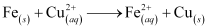But to displace zinc, more reactive metals i.e., metals having lower reduction potentials than zinc such as Mg, Ca, K, etc. are required. But all these metals react with water with the evolution of H2 gas.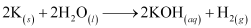As a result, these metals cannot be used in hydrometallurgy to extract zinc.

Hence, copper can be extracted by hydrometallurgy but not zinc.

#### Question 6.2:

What is the role of depressant in froth floatation process?

In the froth floatation process, the role of the depressants is to separate two sulphide ores by selectively preventing one ore from forming froth. For example, to separate two sulphide ores (ZnS and Pbs), NaCN is used as a depressant which selectively allows PbS to come with froth, but prevents ZnS from coming to froth. This happens because NaCN reacts with ZnS to form Na2[Zn(CN)4].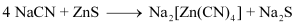#### Question 6.3:

Why is the extraction of copper from pyrites more difficult than that from its

oxide ore through reduction?

The Gibbs free energy of formation (ΔfG) of Cu2S is less than that of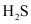and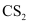. Therefore, H2 and C cannot reduce Cu2S to Cu.

On the other hand, the Gibbs free energy of formation of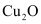is greater than that of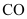. Hence, C can reduce Cu2O to Cu.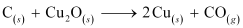Hence, the extraction of copper from its pyrite ore is difficult than from its oxide ore through reduction.

#### Question 6.4:

Explain: (i) Zone refining (ii) Column chromatography.

(i) Zone refining:

This method is based on the principle that impurities are more soluble in the molten state of metal (the melt) than in the solid state. In the process of zone refining, a circular mobile heater is fixed at one end of a rod of impure metal. As the heater moves, the molten zone of the rod also moves with it. As a result, pure metal crystallizes out of the melt and the impurities pass onto the adjacent molten zone. This process is repeated several times, which leads to the segregation of impurities at one end of the rod. Then, the end with the impurities is cut off. Silicon, boron, gallium, indium etc. can be purified by this process.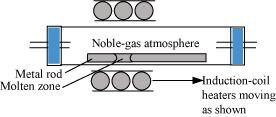(ii) Column chromatography:

Column chromatography is a technique used to separate different components of a mixture. It is a very useful technique used for the purification of elements available in minute quantities. It is also used to remove the impurities that are not very different in chemical properties from the element to be purified. Chromatography is based on the principle that different components of a mixture are differently adsorbed on an adsorbent. In chromatography, there are two phases: mobile phase and stationary phase. The stationary phase is immobile and immiscible. Al2O3 column is usually used as the stationary phase in column chromatography. The mobile phase may be a gas, liquid, or supercritical fluid in which the sample extract is dissolved. Then, the mobile phase is forced to move through the stationary phase. The component that is more strongly adsorbed on the column takes a longer time to travel through it than the component that is weakly adsorbed. The adsorbed components are then removed (eluted) using a suitable solvent (eluant).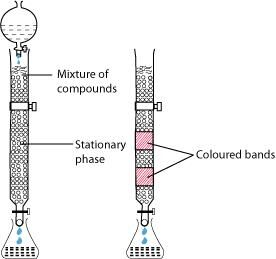#### Question 6.5:

Out of C and CO, which is a better reducing agent at 673 K?

At 673 K, the value of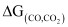is less than that of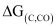. Therefore, CO can be oxidised more easily to CO2 than C to CO. Hence, CO is a better reducing agent than C at 673 K.

#### Question 6.6:

Name the common elements present in the anode mud in electrolytic refining

of copper. Why are they so present ?

In electrolytic refining of copper, the common elements present in anode mud are selenium, tellurium, silver, gold, platinum, and antimony.

These elements are very less reactive and are not affected during the purification process. Hence, they settle down below the anode as anode mud.

#### Question 6.7:

Write down the reactions taking place in different zones in the blast furnace

during the extraction of iron.

During the extraction of iron, the reduction of iron oxides takes place in the blast furnace. In this process, hot air is blown from the bottom of the furnace and coke is burnt to raise the temperature up to 2200 K in the lower portion itself. The temperature is lower in the upper part. Thus, it is the lower part where the reduction of iron oxides (Fe2O3 and Fe3O4) takes place.

The reactions taking place in the lower temperature range (500 − 800 K) in the blast furnace are:

3Fe2O3 + CO→2Fe3O4 + CO2Fe3O4 + 4CO → 3Fe + 4CO2Fe2O3 + CO → 2FeO + CO23Fe2O3 + CO→2Fe3O4 + CO2Fe3O4 + 4CO → 3Fe + 4CO2Fe2O3 + CO → 2FeO + CO2

The reactions taking place in the higher temperature range (900 − 1500 K) in the blast furnace are: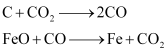The silicate impurity of the ore is removed as slag by calcium oxide (CaO), which is formed by the decomposition of limestone (CaCO3).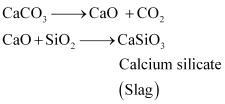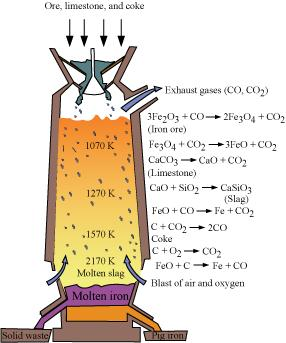#### Question 6.8:

Write chemical reactions taking place in the extraction of zinc from zinc blende.

The different steps involved in the extraction of zinc from zinc blende (ZnS) are given below:

(i) Concentration of ore

First, the gangue from zinc blende is removed by the froth floatation method.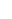(ii) Conversion to oxide (Roasting)

Sulphide ore is converted into oxide by the process of roasting. In this process, ZnS is heated in a regular supply of air in a furnace at a temperature, which is below the melting point of Zn.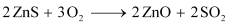(iii) Extraction of zinc from zinc oxide (Reduction)

Zinc is extracted from zinc oxide by the process of reduction. The reduction of zinc oxide is carried out by mixing it with powdered coke and then, heating it at 673 K.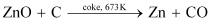(iv) Electrolytic Refining

Zinc can be refined by the process of electrolytic refining. In this process, impure zinc is made the anode while a pure copper strip is made the cathode. The electrolyte used is an acidified solution of zinc sulphate (ZnSO4). Electrolysis results in the transfer of zinc in pure from the anode to the cathode.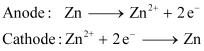#### Question 6.9:

State the role of silica in the metallurgy of copper.

During the roasting of pyrite ore, a mixture of FeO and Cu2O is obtained.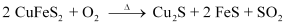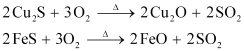The role of silica in the metallurgy of copper is to remove the iron oxide obtained during the process of roasting as ‘slag’. If the sulphide ore of copper contains iron, then silica (SiO2) is added as flux before roasting. Then, FeO combines with silica to form iron silicate, FeSiO3 (slag).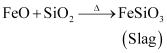#### Question 6.10:

What is meant by the term “chromatography”?

Chromatography is a collective term used for a family of laboratory techniques for the separation of mixtures. The term is derived from Greek words ‘chroma’ meaning ‘colour’ and ‘graphein’ meaning ‘to write’. Chromatographic techniques are based on the principle that different components are absorbed differently on an absorbent. There are several chromatographic techniques such as paper chromatography, column chromatography, gas chromatography, etc.

#### Question 6.11:

What criterion is followed for the selection of the stationary phase in

chromatography?

The stationary phase is selected in such a way that the components of the sample have different solubility’s in the phase. Hence, different components have different rates of movement through the stationary phase and as a result, can be separated from each other.

Question 6.12:

Describe a method for refining nickel.

Nickel is refined by Mond’s process. In this process, nickel is heated in the presence of carbon monoxide to form nickel tetracarbonyl, which is a volatile complex.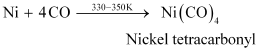Then, the obtained nickel tetracarbonyl is decomposed by subjecting it to a higher temperature (450 − 470 K) to obtain pure nickel metal.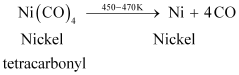#### Question 6.13:

How can you separate alumina from silica in bauxite ore associated with

silica? Give equations, if any.

To separate alumina from silica in bauxite ore associated with silica, first the powdered ore is digested with a concentrated NaOH solution at 473 − 523 K and 35 − 36 bar pressure. This results in the leaching out of alumina (Al2O3) as sodium aluminate and silica (SiO2) as sodium silicate leaving the impurities behind.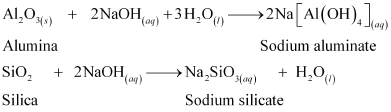Then, CO2 gas is passed through the resulting solution to neutralize the aluminate in the solution, which results in the precipitation of hydrated alumina. To induce precipitation, the solution is seeded with freshly prepared samples of hydrated alumina.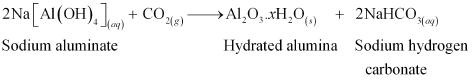During this process, sodium silicate remains in the solution. The obtained hydrated alumina is filtered, dried, and heated to get back pure alumina.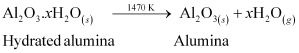#### Question 6.14:

Giving examples, differentiate between ‘roasting’ and ‘calcination’.

Roasting is the process of converting sulphide ores to oxides by heating the ores in a regular supply of air at a temperature below the melting point of the metal. For example, sulphide ores of Zn, Pb, and Cu are converted to their respective oxides by this process.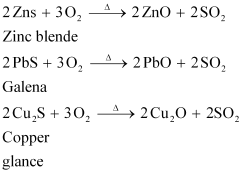On the other hand, calcination is the process of converting hydroxide and carbonate ores to oxides by heating the ores either in the absence or in a limited supply of air at a temperature below the melting point of the metal. This process causes the escaping of volatile matter leaving behind the metal oxide. For example, hydroxide of Fe, carbonates of Zn, Ca, Mg are converted to their respective oxides by this process.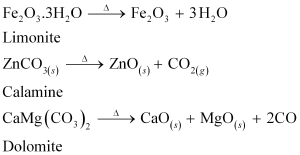#### Question 6.15:

How is ‘cast iron’ different from ‘pig iron”?

The iron obtained from blast furnaces is known as pig iron. It contains around 4% carbon and many impurities such as S, P, Si, Mn in smaller amounts.

Cast iron is obtained by melting pig iron and coke using a hot air blast. It contains a lower amount of carbon (3%) than pig iron. Unlike pig iron, cast iron is extremely hard and brittle.

#### Question 6.16:

Differentiate between “minerals” and “ores”.

Minerals are naturally occurring chemical substances containing metals. They are found in the Earth’s crust and are obtained by mining.

Ores are rocks and minerals viable to be used as a source of metal.

For example, there are many minerals containing zinc, but zinc cannot be extracted profitably (conveniently and economically) from all these minerals.

Zinc can be obtained from zinc blende (ZnS), calamine (ZnCO3), Zincite (ZnO) etc.

Thus, these minerals are called ores of zinc.

#### Question 6.17:

Why copper matte is put in silica lined converter?

Copper matte contains Cu2S and FeS. Copper matte is put in a silica-lined converter to remove the remaining FeO and FeS present in the matte as slag (FeSiO3). Also, some silica is added to the silica-lined converter. Then, a hot air blast is blown. As a result, the remaining FeS and FeO are converted to iron silicate (FeSiO3) and Cu2S is converted into metallic copper.#### Question 6.18:

What is the role of cryolite in the metallurgy of aluminium?

Cryolite (Na3AlF6) has two roles in the metallurgy of aluminium:

1. To decrease the melting point of the mixture from 2323 K to 1140 K.

2. To increase the electrical conductivity of Al2O3.

#### Question 6.19:

How is leaching carried out in case of low grade copper ores?

In case of low grade copper ores, leaching is carried out using acid or bacteria in the presence of air. In this process, copper goes into the solution as Cu2+ ions.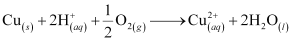The resulting solution is treated with scrap iron or H2 to get metallic copper.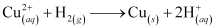#### Question 6.20:

Why is zinc not extracted from zinc oxide through reduction using CO?

The standard Gibbs free energy of formation of ZnO from Zn

is lower than that of CO2 from CO. Therefore, CO cannot reduce ZnO to Zn. Hence, Zn is not extracted from ZnO through reduction using CO.

#### Question 6.21:

The value of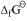for formation of Cr2Ois − 540 kJmol−1 and that of Al2 O3 is − 827 kJmol−1. Is the reduction of Cr2O3 possible with Al?

The value offor the formation of Cr2O3 from Cr (−540 kJmol−1) is higher than that of Al2O3 from Al (−827 kJmol−1). Therefore, Al can reduce Cr2O3 to Cr. Hence, the reduction of Cr2O3 with Al is possible.

Alternatively,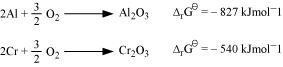Subtracting equation (ii) from (i), we have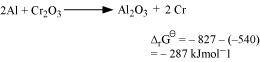Asfor the reduction reaction of Cr2O3 by Al is negative, this reaction is possible.

#### Question 6.22:

Out of C and CO, which is a better reducing agent for ZnO ?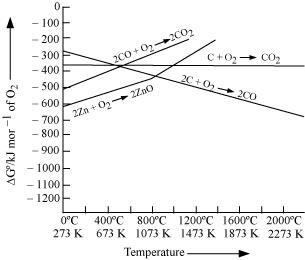Reduction of ZnO to Zn is usually carried out at 1673 K. From the above figure, it can be observed that above 1073 K, the Gibbs free energy of formation of CO from C and above 1273 K, the Gibbs free energy of formation of CO2 from C is lesser than the Gibbs free energy of formation of ZnO. Therefore, C can easily reduce ZnO to Zn.

On the other hand, the Gibbs free energy of formation of CO2 from CO is always higher than the Gibbs free energy of formation of ZnO. Therefore, CO cannot reduce ZnO. Hence, C is a better reducing agent than CO for reducing ZnO.

#### Question 6.23:

The choice of a reducing agent in a particular case depends on

thermodynamic factor. How far do you agree with this statement? Support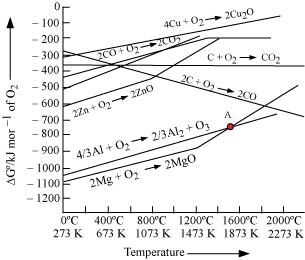The above figure is a plot of Gibbs energy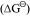vs. T for formation of some oxides.

It can be observed from the above graph that a metal can reduce the oxide of other metals, if the standard free energy of formation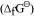of the oxide of the former is more negative than the latter. For example, since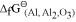is more negative than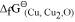, Al can reduce Cu2O to Cu, but Cu cannot reduce Al2O3. Similarly, Mg can reduce ZnO to Zn, but Zn cannot reduce MgO because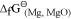is more negative than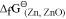.

#### Question 6.24:

Name the processes from which chlorine is obtained as a by-product. What

will happen if an aqueous solution of NaCl is subjected to electrolysis?

In the electrolysis of molten NaCl, Cl2 is obtained at the anode as a by product.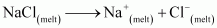At cathode: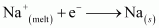At anode: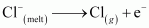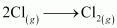The overall reaction is as follows: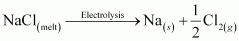If an aqueous solution of NaCl is electrolyzed, Cl2 will be obtained at the anode but at the cathode, H2 will be obtained (instead of Na). This is because the standard reduction potential of Na (E°= − 2.71 V) is more negative than that of H2O (E° = − 0.83 V). Hence, H2O will get preference to get reduced at the cathode and as a result, H2 is evolved.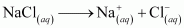At cathode: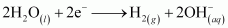At anode:#### Question 6.25:

What is the role of graphite rod in the electrometallurgy of aluminium?

In the electrometallurgy of aluminium, a fused mixture of purified alumina (Al2O3), cryolite (Na3AlF6) and fluorspar (CaF2) is electrolysed. In this electrolysis, graphite is used as the anode and graphite-lined iron is used as the cathode. During the electrolysis, Al is liberated at the cathode, while CO and CO2 are liberated at the anode, according to the following equation.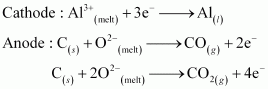If a metal is used instead of graphite as the anode, then O2 will be liberated. This will not only oxidise the metal of the electrode, but also convert some of the Al liberated at the cathode back into Al2O3. Hence, graphite is used for preventing the formation of O2 at the anode. Moreover, graphite is cheaper than other metals.

#### Question 6.27:

Outline the principles of refining of metals by the following methods:

(i) Zone refining

(ii) Electrolytic refining

(iii) Vapour phase refining

(i) Zone refining:

This method is based on the principle that impurities are more soluble in the molten state of metal (the melt) than in the solid state. In the process of zone refining, a circular mobile heater is fixed at one end of a rod of impure metal. As the heater moves, the molten zone of the rod also moves along with it. As a result, pure metal crystallizes out of the melt and the impurities pass to the adjacent molten zone. This process is repeated several times, which leads to the segregation of impurities at one end of the rod. Then, the end with the impurities is cut off. Silicon, boron, gallium, indium etc. can be purified by this process.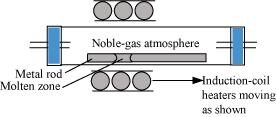(ii) Electrolytic refining;

Electrolytic refining is the process of refining impure metals by using electricity. In this process, impure metal is made the anode and a strip of pure metal is made the cathode. A solution of a soluble salt of the same metal is taken as the electrolyte. When an electric current is passed, metal ions from the electrolyte are deposited at the cathode as pure metal and the impure metal from the anode dissolves into the electrolyte in the form of ions. The impurities present in the impure metal gets collected below the anode. This is known as anode mud.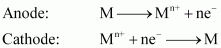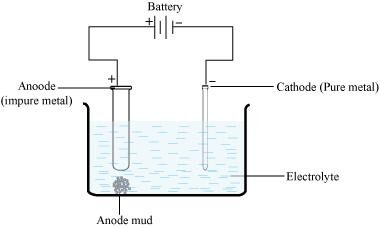(iii) Vapour phase refining

Vapour phase refining is the process of refining metal by converting it into its volatile compound and then, decomposing it to obtain a pure metal. To carry out this process,

(i) the metal should form a volatile compound with an available reagent, and

(ii) the volatile compound should be easily decomposable so that the metal can be easily recovered.

Nickel, zirconium, and titanium are refined using this method.

#### Question 6.28:

Predict conditions under which Al might be expected to reduce MgO.